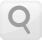# CASIO

User´s Guide## Calculation Priority Sequence

The calculator performs calculations according to a calculation priority sequence.

When the priority of two expressions is the same, the calculation is performed from left to right.

1 Function with parentheses: Pol(x, y), Rec(r, θ), RanInt#(a, b)* (*fx-220 PLUS only) Type A functions: With these functions, the value is entered and then the function key is pressed. (x3, x2, x-1, x!, ° ’ ”, xˆ, xˆ1, xˆ2, yˆ, °, r, g) Powers and roots: xy, x√ Fractions Implied multiplication of π, e (natural logarithm base), memory name, or variable name: 2π, 3e, 5A, πA, etc. Type B functions: With these functions, the function key is pressed and then the value is entered. (√ , 3√ , log, ln, ex, 10x, sin, cos, tan, sin-1, cos-1, tan-1, sinh, cosh, tanh, sinh-1, cosh-1, tanh-1, (-)) Implied multiplication of Type B functions: 2√3, Alog2, etc. Permutation (nPr), combination (nCr) Multiplication, division (×, ÷) Addition, subtraction (+, -)

The negative sign (-) is treated as a Type B function, so particular care is required when the calculation includes a high-priority Type A function, or power or root operations.

Example: (-2)4 = 16; -24 = -16